Popular tags: Support, Resistance, Trend, Range, Fibonacci, Wave, Divergence, Multi time frames(MTF), Swing, Volume, Overbought, Oversold

Featured

# Auto ZigZag Fibonacci extension indicator Ninjatrader 8

Availability: In Stock
Price: \$149.00USD \$120.00USD
Qty:     - OR -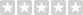(0/5)  0 reviews  |  Write a review

VIP price: \$100  This is a permanent license.

Fibonacci retracement is a method of technical analysis for determining support and resistance levels. They are named after their use of the Fibonacci sequence. Fibonacci retracement is based on the idea that markets will retrace a predictable portion of a move, after which they will continue to move in the original direction.

This custom Ninjatrader indicator will plot Fibonacci extension lines automatically from previous Zigzag High or Low.
It works on any timeframe and chart type that supports ZigZag, such as Renko, Heiken Ashi, Range and Tick.

It uses the built-in version of Zigzag from NT8, but instead of plotting Zigzag on chart, it draws the Fibonacci extension lines.

There are two Zigzag deviation type:  percentage and point. The parameter "deviation value" is the actual number used for deviation type.
When you use percentage as the deviation type, it means that the value difference between two adjacent Zigzag point must be greater than the input percentage  which is the deviation value.

For example, if you used percentage deviation type and set deviation value to 0.1, then the value difference between each Zigzag point must greater than 0.1%. It also applies to all adjacent Zigzag points.

When you use point as the deviation type, it means that the value difference between two adjacent Zigzag point must be greater than the input point which is the deviation value. The value of Point is the actual price amount, if you set deviation value to 1, the value difference between each Zigzag point must greater than \$1. It also applies to all adjacent Zigzag points.

For example, if you used percentage deviation type and set deviation value to 0.1, the value difference between two adjacent Zigzag points must greater than \$0.1.It has all the inputs of Zigzag and two additional inputs for Fibonacci extension, if you checked "Show Extra lines", it will plot the Fibonacci retracement of 0.236, 0.382 and 0.618 above and below the 0% and 100%.

If "Use Recent ZigZag" is enabled, the indicator will use the most recent stable ZigZag, otherwise it will use the previous one. Please check the images below for a better understanding.The indicator may not work properly if there was not enough bars on the chart.

In general, 800 bars is good, but it also depends on the ZigZag Deviation value you set.

The bigger the Deviation value is the more bars you need to load.

You can change "bars to load" by following the pic below.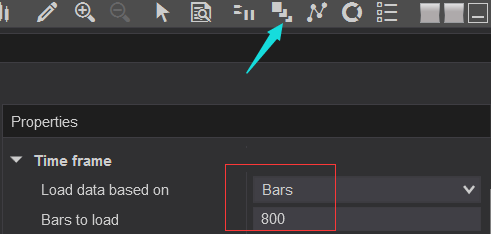The price percentage change of Stock, Forex and Futures vary widely in the same timeframe.

In most case, Stocks have higher percentage change than Forex and Futures.

The fastest way to access your fav inputs is saving it as Templates.

Deviation percent value 0.01-0.065 is good for Forex in 1 to 5 minutes chart, but it may not work well for stocks or futures.

Please check the screenshots below to get some ideas of how to properly set the inputs.

Example of Heiken Ashi:Example of Renko: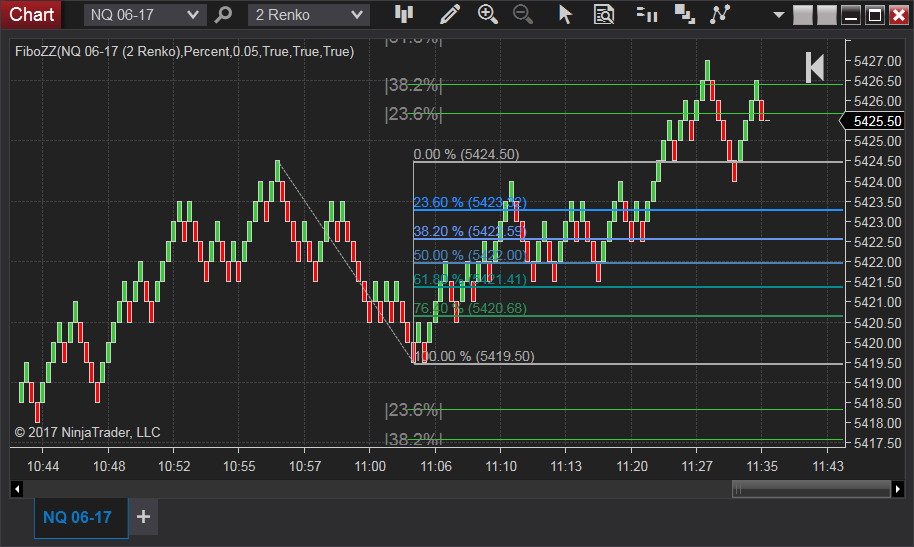Example of Tick.

Example of  Range: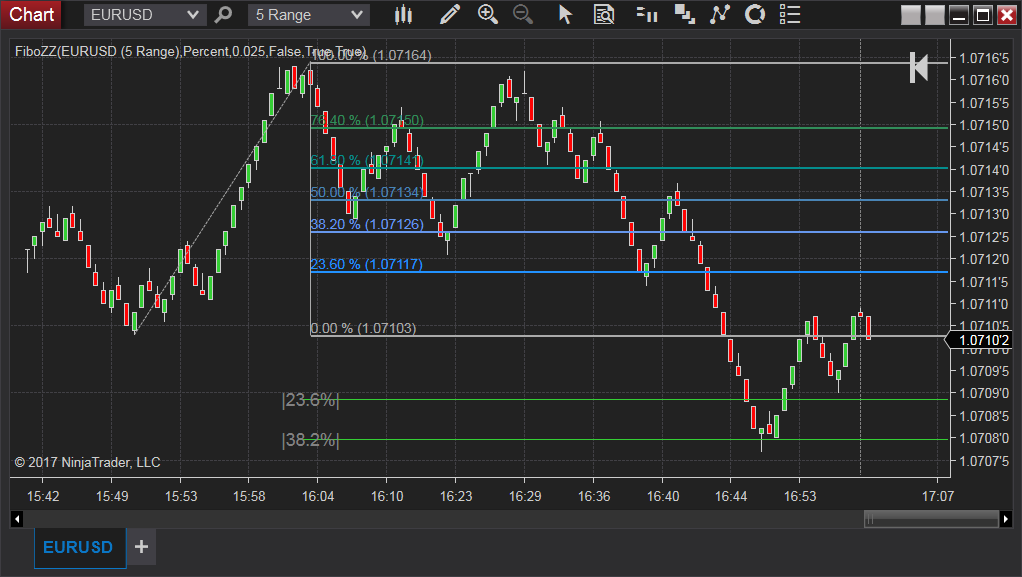Example of minutes: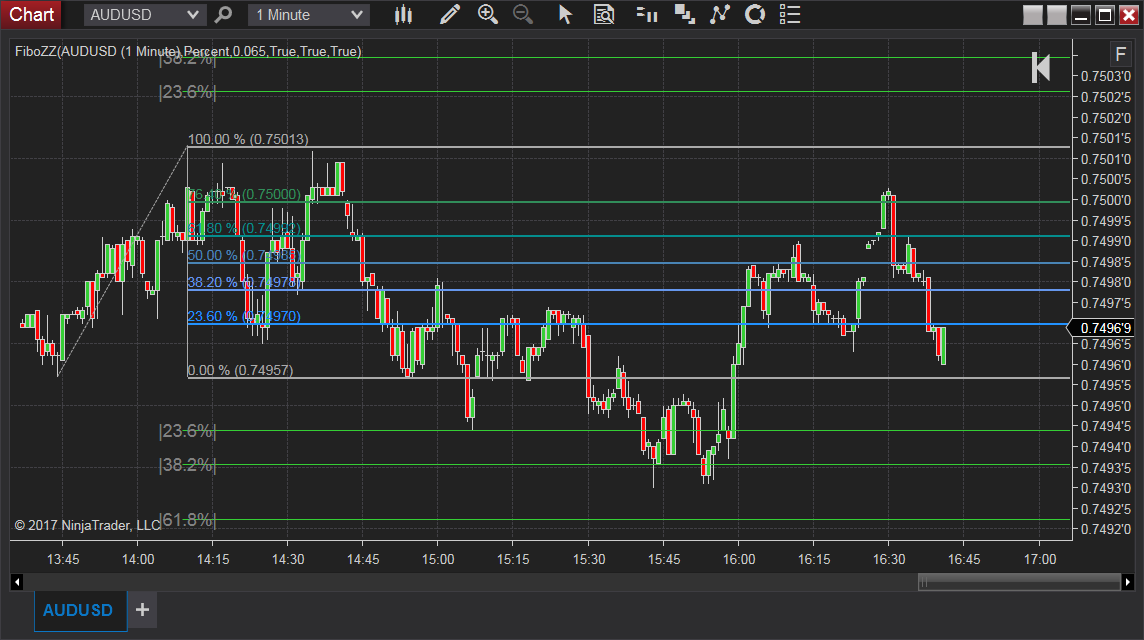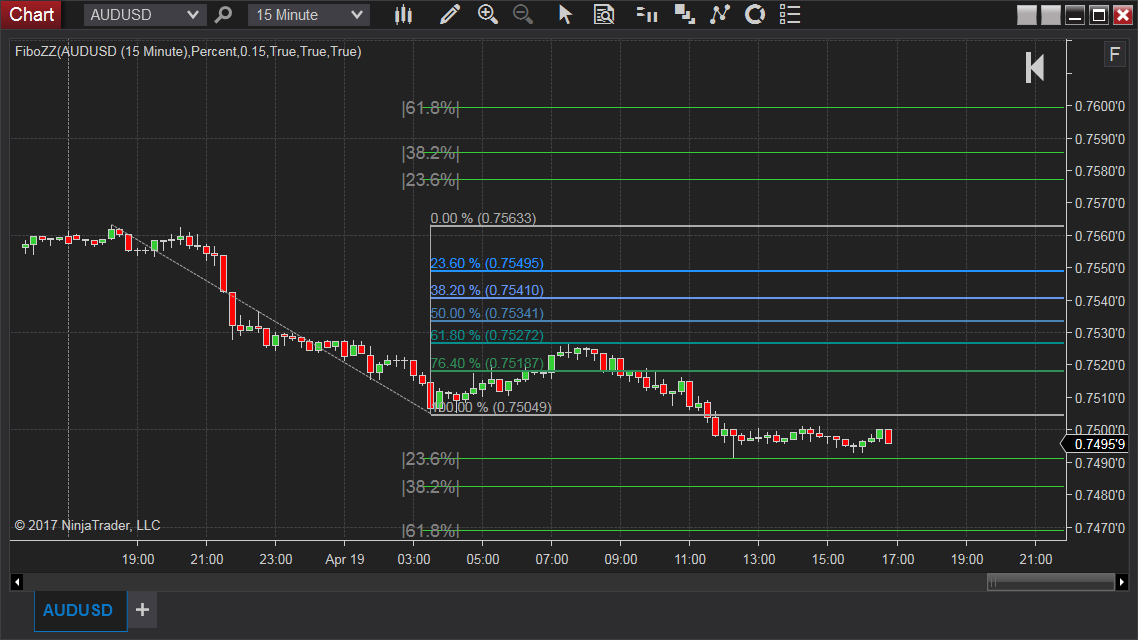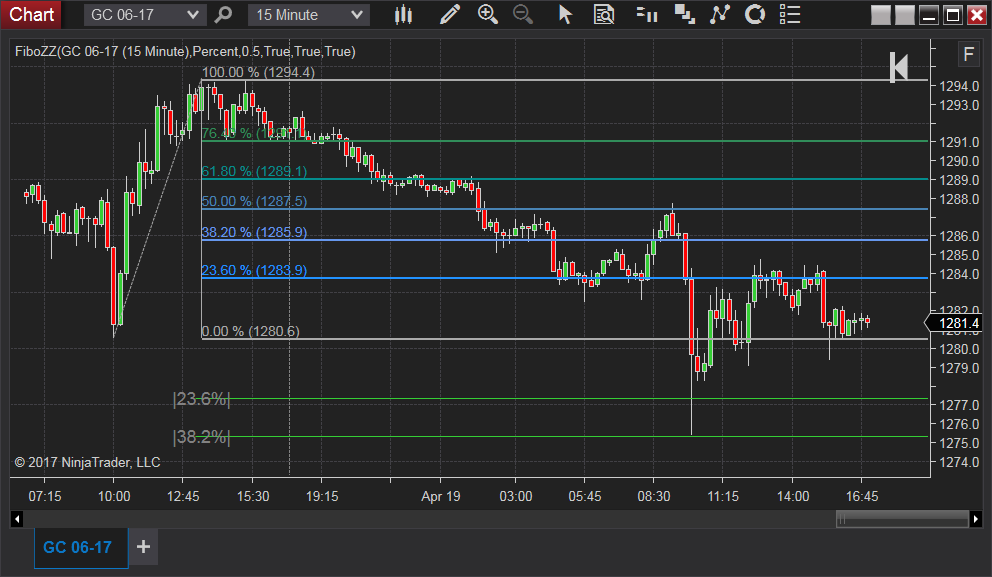## Write a review

Your Review: Note: HTML is not translated!

Enter the code in the box below:Tags: zigzag, fibo
Latest
Welcome to Patternsmart
Converting your indicator from one platform to another.